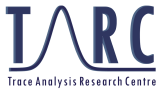# Trace Analysis Research Centre

## Department of Chemistry

.

### Educational Material

One of the ways in which members of TARC have contributed to advances in the science of chemical measurement is the preparation of videos on various related topics. These topics include teaching online, scientific writing, methods of research, and various aspects of mass spectrometry and proteomics. These videos are posted to a YouTube Channel for viewing.

Another method of contribution is through the development of textual material. This started as a simple text, A Brief Introduction to Analytical Chemistry, which originated from the notes for a one-semester introductory course in chemical analysis. The topics covered included statistics, stoichiometry, ionic equilibria, introduction to instrumental analysis, spectroscopy, redox and electrochemistry, and chromatography. This original text has been expanded, enlarged, and produced in a much more professional version as An Introduction to Analytical Chemistry. The material in these texts is presented at a level that assumes that the reader has taken an introductory university chemistry course and is suitable for both chemistry majors and non-majors, e.g. biologists or geologists. The approach is approximately half classical, including material on equilibria and titrations, and half instrumental, including material on instrumental methods commonly found in most laboratories.

### A Brief Introduction to Analytical Chemistry

Chapter 1. Introductory Material
1.1 Statistical treatment of data
1.2 Propagation of errors in calculations
1.3 Linear least squares curve fitting
1.4 Quantitative calculations involving concentration
1.5 Quantitative calculations involving titrations
Chapter 2. Ionic Equilibria
2.1 Behaviour of ionic solutions
2.2 Acid-base reactions
2.3 General method for the solution of equilibrium problems
2.4 Equilibria involving acids and bases
2.5 Acid-base mixtures
2.6 Theory of titrations
2.7 Indicators in acid-base titrations
2.8 Buffer solutions
2.9 Polyfunctional acids and bases
Chapter 3. Introduction to Instrumental Methods
3.1 Instrumental response
3.2 Relating response to concentration
3.3 Detection limits
Chapter 4. Spectroscopy
4.1 The interaction of light and matter
4.2 Absorption spectroscopy in solution
4.3 Molecular emission spectroscopy
4.4 Atomic spectroscopy
Chaprter 5. Redox Reactions and Electrochemistry
5.1 Introduction
5.2 Calculation of cell voltages
5.3 Half cell method of voltage calculation
5.4 Redox titrations
5.5 Potentiometry
Chapter 6. Chromatography
6.1 Introduction
6.2 Theory of chromatography
6.3 Types of chromatography
6.4 Uses of chromatography
Appendix A. Supplemental Material
Quadratic formula, use of calculators, balancing redox reactions, electrochemical calculations
Appendix B. Tables
Atomic masses, statistical parameters, ion size parameters, dissociation constants, standard potentials